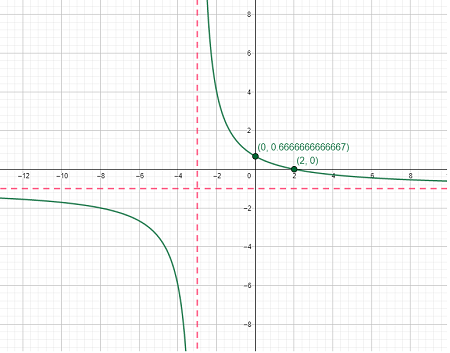# Draw the graph for the following function: f(x)= \frac{2-x}{3+x}

## Question:

Draw the graph for the following function: {eq}f(x)= \frac{2-x}{3+x} {/eq}

## Graphing Rational Functions with Calculus:

To graph a rational function {eq}\dfrac{p(x)}{q(x)} {/eq} where {eq}p(x), q(x) {/eq} are polynomials using calculus, follow the steps below

• Identify any asymptotes of the function
• Find any x or y intercepts of the function
• Determine where the function is increasing or decreasing by using the first derivative and finding critical points
• Determine where the function is concave up or concave down by using the second derivative and finding inflection points
• Plot all asymptotes and points found above and connect with a smooth curve following the information about increasing/decreasing and concavity

First, find any asymptotes of {eq}f(x) = \dfrac{2-x}{3+x} {/eq}. The function has a vertical asymptote where it is undefined, which is where

{eq}3+x=0\\ x=-3 {/eq}

To find any horizontal asymptotes, find the limit as x approaches positive or negative infinity

{eq}\lim\limits_{x\to\pm\infty}\dfrac{2-x}{3+x} = -1 {/eq} since the degrees of the polynomials in the numerator and denominator are equal, but the leading terms are of opposite sign. So we have a horizontal asymptote of {eq}y=-1 {/eq}

To find any intercepts of the function, start with the y-intercept. Simply substitute x=0 into the function

{eq}f(0) = \dfrac{2-0}{3+0} = \dfrac{2}{3} {/eq} so the y-intercept is {eq}\left(0,\frac{2}{3}\right) {/eq}

The x-intercept(s) occur when {eq}f(x) = 0 {/eq} so we have {eq}\dfrac{2-x}{3+x} = 0\\ 2-x=0\\ 2=x {/eq} and so the x-intercept is {eq}(2,0) {/eq}

Now find where the function is increasing or decreasing by taking the derivative with the quotient rule and finding critical points (where the derivative is undefined or equal to zero)

{eq}f'(x) = \dfrac{(3+x)(-1) - (2-x)(1)}{(3+x)^2}\\ f'(x) = \dfrac{-3-x-2+x}{(3+x)^2}\\ f'(x) = \dfrac{-5}{(3+x)^2} {/eq}

The derivative is never zero, since in order for the derivative to be zero, the numerator would need to equal zero. The derivative is undefined when {eq}x=-3 {/eq}, however this is not a critical point since it corresponds to our vertical asymptote. Checking values on either side of the vertical asymptote in the derivative, we have

{eq}f'(-4) = \dfrac{-5}{(3-4)^2} = \dfrac{-5}{1} <0\\ f'(-2) = \dfrac{-5}{(3-2)^2} = \dfrac{-5}{1} <0 {/eq}

So the function is decreasing on {eq}(-\infty, -3)\cup (-3,\infty) {/eq} and never increasing.

Now find the concavity of the function by finding the second derivative and inflection points (where the second derivative is zero or undefined). Using the quotient rule,

{eq}f'(x) = \dfrac{-5}{(3+x)^2}\\ f''(x) = \dfrac{(3+x)^2(0) -(-5)(2(3+x)(1))}{(3+x)^4}\\ f''(x) = \dfrac{10(3+x)}{(3+x)^4}\\ f''(x) = \dfrac{10}{(3+x)^3} {/eq}

The second derivative is never zero since its numerator is never zero. The second derivative is undefined when {eq}x=-3 {/eq}, but this is not an inflection point since it is the vertical asymptote again. Checking values on either side of the vertical asymptote, we have

{eq}f''(-4) = \dfrac{10}{(3-4)^3} = \dfrac{10}{-1} <0\\ f''(-2) = \dfrac{10}{(3-2)^3} = \dfrac{10}{1} >0 {/eq}

The function is concave up on {eq}(-3,\infty) {/eq} and concave down on {eq}(-\infty, - 3) {/eq}

Plotting all asymptotes, intercepts, and using the information about concavity and increasing/decreasing, we have